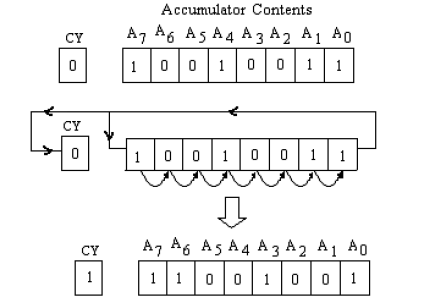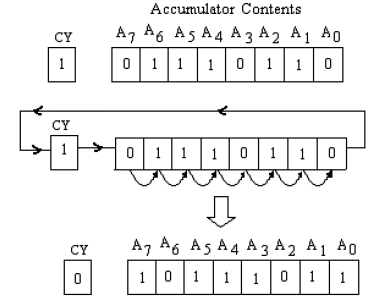# RRC and RAR in 8085

Last updated on May 17th, 2022 at 04:18 pm

In this post, we will see with examples how RRC and RAR instructions in 8085 work and how they differ in their operations.

Instruction RRC and RAR are basically two logical instructions available in instruction sets of 8085, to rotate the bits of the accumulator one position to the right.

## Define RRC and RAR instructions

RRC: Rotate the accumulator right. Bit 0 (LSB) goes to both Bit 7(MSB) and the Carry Flag (CY).

RAR: Rotate the accumulator right through the carry. Bit 0 goes to the Carry Flag (CY) and carry goes to Bit 7.

## Differences between RRC and RAR instructions in 8085

In RRC the accumulator is rotated to the right, and as a result Bit 0 (LSB) goes to both Bit 7(MSB) and the Carry Flag (CY). In RAR the accumulator is rotated to the right through the carry, and as a result Bit 0 goes to the Carry Flag (CY) and carry goes to Bit 7.

Now to understand the difference between these two instructions, we will take help of a numerical example and diagrams.

## How RRC functions – show with a numerical example

Question 1) What will be the content of the accumulator and the status of CY flag after RRC operation, if the content of the accumulator is 93H and the content of CY is 0?

Solution:

Content of accumulator (ACC) is 93H= (1001 0011)2 and CY=0

Now content of the accumulator and the carry flag is shown below with the rotate right operation, before and after execution of RRC instruction for single time.

In this instruction, all the bits of accumulator are shifted or rotated right.

The content of LSB will be stored in the MSB of the accumulator as well as in the CY flag.Figure1: Content of the accumulator and carry flag before and after the execution of RRC instruction.

Now after execution of instruction RRC one time, the content of accumulator (ACC) is (1100 1001)2= C9H and the Carry flag is SET i.e. CY=1

In this instruction only carry flag CY will be affected and all other flags will be unaffected.

## How RAR functions – show with a numerical example

Question 2) What will be the content of the accumulator and the status of CY flag after RAR operation, if the content of the accumulator is 76H and the content of CY is 1?

Solution:

Content of accumulator (ACC) is 76H= (0111 0110)2 and CY=1, before the execution of RAR instruction. Then after the execution of this instruction the accumulator contents will be shifted as shown in figure below.

In this rotate instruction, all the bits of the accumulator contents will be shifted /rotated right through carry.

The content of carry flag CY will be stored in MSB of the accumulator and LSB of the accumulator will be stored in CY flag; and all other bits of the accumulator will be shifted to the right.Figure 2 – content of the accumulator and carry flag before and after execution of RAR instruction.

Now after execution of instruction RAR one time, the content of accumulator (ACC) is (1011 1011)2= BBH and the Carry flag is RESET i.e. CY=0

In this instruction too only carry flag will be affected.